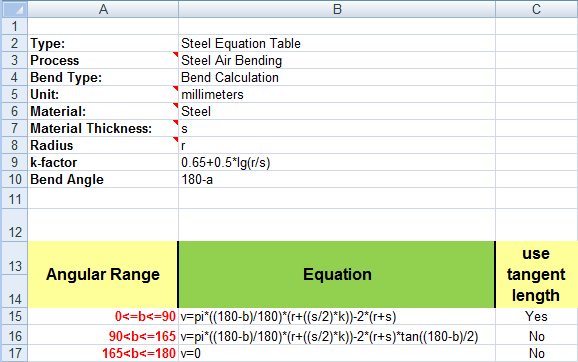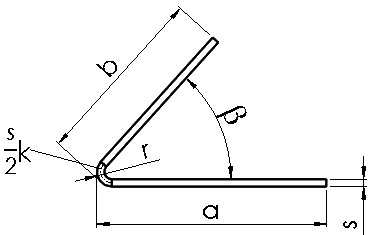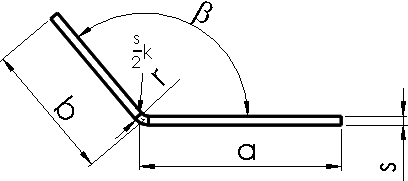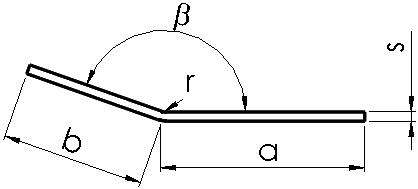Bend Allowance and Bend Deduction OptionsBend Allowance and Bend DeductionK-factorBend Calculation TablesBend Table OverviewSheet Metal Default Bend Parameters

Bend Calculation Tables

You can calculate the developed length of sheet metal parts using bend calculation tables.

With bend calculation tables, you can define different angular ranges, assign equations to those ranges, and calculate the developed length of the part.

The default location for bend calculation tables is install_dir\lang\english\Sheetmetal Bend Tables.

Example of a bend calculation table:Column A Displays the variables that can be used in equations:
• s = material thickness
• r = bend radius
• k-factor = k-factor (you can use an equation or a value)
• ß = aperture angle
Angular Range Lets you define the angular ranges. The angular range defines the different ranges that must be used for specific calculations.
• You define the smallest angular value of a range to the left of ß
• ß = angle variable
• You define the largest angular value of a range to the right of ß
Equation Lets you define the equation to use for the angular range. You can also use a constant value.
use tangent length Lets you determine the method to calculate flanges: virtual sharp or tangent length.

The equation that calculates developed length is: LD = A + B + V, where V can be negative. When V is negative, it is the same as using bend deduction, even though the equation is for bend allowance.

Angular Range Example
0 <= ß <= 90 Tangent length = Yes90 < ß <= 165 Tangent length = No165 < ß <= 180 Tangent length = NoProvide feedback on this topic

SOLIDWORKS welcomes your feedback concerning the presentation, accuracy, and thoroughness of the documentation. Use the form below to send your comments and suggestions about this topic directly to our documentation team. The documentation team cannot answer technical support questions. Click here for information about technical support.

* Required

 *Email: Subject: Feedback on Help Topics Page: Bend Calculation Tables *Comment: * I acknowledge I have read and I hereby accept the privacy policy under which my Personal Data will be used by Dassault Systèmes

Print Topic

Select the scope of content to print:

x

We have detected you are using a browser version older than Internet Explorer 7. For optimized display, we suggest upgrading your browser to Internet Explorer 7 or newer.

Never show this message again
x

Web Help Content Version: SOLIDWORKS 2012 SP05

To disable Web help from within SOLIDWORKS and use local help instead, click Help > Use SOLIDWORKS Web Help.

To report problems encountered with the Web help interface and search, contact your local support representative. To provide feedback on individual help topics, use the “Feedback on this topic” link on the individual topic page.